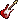» » »

# Chapman Tracy - Baby Can I Hold You Tabs

Artists:  A B C D E F G H I J K L M N O P Q R S T U V W X Y Z #

## Baby Can I Hold You Tabs

Chapman Tracy

```Tabs too difficult? Try these video lessons and learn fast
```
```                {title:Baby Can I Hold You}
{st:by Tracy CHAPMAN}
D                 A7sus   A7
e=====2===2==2=0===2===|0===0===0========
B==T==3===3==3=3===3=0=|3===3=0=2========
G==A==2===2==2=2===2===|0===0===0========
D==B==0===0==0=0===0===|2===2===2========
A======================|0===0===0========
E======================|=================
Sorry[D]
[A7sus]Is all that [A7]you can't s[Em]ay
Y[A7sus]ears gone by[A7] and st[D]ill
[A7sus]Words don't c[A7]ome easil[Em]y
[G]Like so[A]rry [G]like so[A]rry
Forgive me
Is all that you can't say
Years gone by and still
Words don't come easily
Like forgive me forgive me
{c:chorus}
But you can say baby
[Em]baby, can I [G]hold you [D]tonight.
[Em]Baby, if I [G]told you the [Bm]right words,
oo= at the righ[A]t time, you'd be mine.[D]  === [Em] === [F#m] === [G] === [A] repeat
I love you
Is all that you can't say
Years gone by and still
Words don't come easily
Like I love you I love you
{c: to chorus}
```
`Submit corrections`

```Hard to play? Try these video lessons and learn fast```
↑ Back to top | Tablatures and chords for acoustic guitar and electric guitar, ukulele, drums are parodies/interpretations of the original songs. You may use it for private study, scholarship, research or language learning purposes only